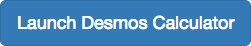## Rules for Exponents

### Learning Outcomes

• Use the product rule for exponents.
• Use the quotient rule for exponents.
• Use the power rule for exponents.Consider the product ${x}^{3}\cdot {x}^{4}$. Both terms have the same base, x, but they are raised to different exponents. Expand each expression, and then rewrite the resulting expression.

\begin{align}x^{3}\cdot x^{4}&=\stackrel{\text{3 factors }}{(x\cdot x\cdot x)} \stackrel{\text{ 4 factors}}{(x\cdot x\cdot x\cdot x)} \\ & =\stackrel{7 \text{ factors}}{x\cdot x\cdot x\cdot x\cdot x\cdot x\cdot x} \\ & =x^{7}\end{align}

The result is that ${x}^{3}\cdot {x}^{4}={x}^{3+4}={x}^{7}$.

Notice that the exponent of the product is the sum of the exponents of the terms. In other words, when multiplying exponential expressions with the same base, we write the result with the common base and add the exponents. This is the product rule of exponents.

${a}^{m}\cdot {a}^{n}={a}^{m+n}$

Now consider an example with real numbers.

${2}^{3}\cdot {2}^{4}={2}^{3+4}={2}^{7}$

We can always check that this is true by simplifying each exponential expression. We find that ${2}^{3}$ is 8, ${2}^{4}$ is 16, and ${2}^{7}$ is 128. The product $8\cdot 16$ equals 128, so the relationship is true. We can use the product rule of exponents to simplify expressions that are a product of two numbers or expressions with the same base but different exponents.

### A General Note: The Product Rule of Exponents

For any real number $a$ and natural numbers $m$ and $n$, the product rule of exponents states that

${a}^{m}\cdot {a}^{n}={a}^{m+n}$

### Example: Using the Product Rule

Write each of the following products with a single base. Do not simplify further.

1. ${t}^{5}\cdot {t}^{3}$
2. $\left(-3\right)^{5}\cdot \left(-3\right)$
3. ${x}^{2}\cdot {x}^{5}\cdot {x}^{3}$

### Try It

Write each of the following products with a single base. Do not simplify further.

1. ${k}^{6}\cdot {k}^{9}$
2. ${\left(\dfrac{2}{y}\right)}^{4}\cdot \left(\dfrac{2}{y}\right)$
3. ${t}^{3}\cdot {t}^{6}\cdot {t}^{5}$

In the following video we show more examples of how to use the product rule to simplify an expression with exponents.

## Using the Quotient Rule of Exponents

The quotient rule of exponents allows us to simplify an expression that divides two numbers with the same base but different exponents. In a similar way to the product rule, we can simplify an expression such as $\dfrac{{y}^{m}}{{y}^{n}}$, where $m>n$. Consider the example $\dfrac{{y}^{9}}{{y}^{5}}$. Perform the division by canceling common factors.

\begin{align}\frac{y^{9}}{y^{5}} &=\frac{y\cdot y\cdot y\cdot y\cdot y\cdot y\cdot y}{y\cdot y\cdot y\cdot y\cdot y} \\[1mm] &=\frac{\cancel{y}\cdot\cancel{y}\cdot\cancel{y}\cdot\cancel{y}\cdot\cancel{y}\cdot y\cdot y\cdot y\cdot y}{\cancel{y}\cdot\cancel{y}\cdot\cancel{y}\cdot\cancel{y}\cdot\cancel{y}} \\[1mm] & =\frac{y\cdot y\cdot y\cdot y}{1} \\[1mm] & =y^{4}\\ \text{ }\end{align}

Notice that the exponent of the quotient is the difference between the exponents of the divisor and dividend.

$\dfrac{{a}^{m}}{{a}^{n}}={a}^{m-n}$

In other words, when dividing exponential expressions with the same base, we write the result with the common base and subtract the exponents.

$\dfrac{{y}^{9}}{{y}^{5}}={y}^{9 - 5}={y}^{4}$

For the time being, we must be aware of the condition $m>n$. Otherwise, the difference $m-n$ could be zero or negative. Those possibilities will be explored shortly. Also, instead of qualifying variables as nonzero each time, we will simplify matters and assume from here on that all variables represent nonzero real numbers.

### A General Note: The Quotient Rule of Exponents

For any real number $a$ and natural numbers $m$ and $n$, such that $m>n$, the quotient rule of exponents states that

$\dfrac{{a}^{m}}{{a}^{n}}={a}^{m-n}$

### Example: Using the Quotient Rule

Write each of the following products with a single base. Do not simplify further.

1. $\dfrac{{\left(-2\right)}^{14}}{{\left(-2\right)}^{9}}$
2. $\dfrac{{t}^{23}}{{t}^{15}}$
3. $\dfrac{{\left(z\sqrt{2}\right)}^{5}}{z\sqrt{2}}$

### Try It

Write each of the following products with a single base. Do not simplify further.

1. $\dfrac{{s}^{75}}{{s}^{68}}$
2. $\dfrac{{\left(-3\right)}^{6}}{-3}$
3. $\dfrac{{\left(e{f}^{2}\right)}^{5}}{{\left(e{f}^{2}\right)}^{3}}$

Watch this video to see more examples of how to use the quotient rule for exponents.

## Using the Power Rule of Exponents

Suppose an exponential expression is raised to some power. Can we simplify the result? Yes. To do this, we use the power rule of exponents. Consider the expression ${\left({x}^{2}\right)}^{3}$. The expression inside the parentheses is multiplied twice because it has an exponent of 2. Then the result is multiplied three times because the entire expression has an exponent of 3.

\begin{align} {\left({x}^{2}\right)}^{3}& = \stackrel{{3\text{ factors}}}{{{\left({x}^{2}\right)\cdot \left({x}^{2}\right)\cdot \left({x}^{2}\right)}}} \\ & = \stackrel{{3\text{ factors}}}{\overbrace{{\left(\stackrel{{2\text{ factors}}}{{\overbrace{x\cdot x}}}\right)\cdot \left(\stackrel{{2\text{ factors}}}{{\overbrace{x\cdot x}}}\right)\cdot \left(\stackrel{{2\text{ factors}}}{{\overbrace{x\cdot x}}}\right)}}}\\ & = x\cdot x\cdot x\cdot x\cdot x\cdot x\hfill \\ & = {x}^{6} \end{align}

The exponent of the answer is the product of the exponents: ${\left({x}^{2}\right)}^{3}={x}^{2\cdot 3}={x}^{6}$. In other words, when raising an exponential expression to a power, we write the result with the common base and the product of the exponents.

${\left({a}^{m}\right)}^{n}={a}^{m\cdot n}$

Be careful to distinguish between uses of the product rule and the power rule. When using the product rule, different terms with the same bases are raised to exponents. In this case, you add the exponents. When using the power rule, a term in exponential notation is raised to a power. In this case, you multiply the exponents.

Product Rule Power Rule
$5^{3}\cdot5^{4}$ =  $5^{3+4}$ = $5^{7}$ but $\left(5^{3}\right)^{4}$ = $5^{3\cdot4}$ = $5^{12}$
$x^{5}\cdot x^{2}$ = $x^{5+2}$ = $x^{7}$ but $\left(x^{5}\right)^{2}$ =  $x^{5\cdot2}$ = $x^{10}$
$\left(3a\right)^{7}\cdot\left(3a\right)^{10}$ = $\left(3a\right)^{7+1-}$ = $\left(3a\right)^{17}$ but $\left(\left(3a\right)^{7}\right)^{10}$ = $\left(3a\right)^{7\cdot10}$ = $\left(3a\right)^{70}$

### A General Note: The Power Rule of Exponents

For any real number $a$ and positive integers $m$ and $n$, the power rule of exponents states that

${\left({a}^{m}\right)}^{n}={a}^{m\cdot n}$

### Example: Using the Power Rule

Write each of the following products with a single base. Do not simplify further.

1. ${\left({x}^{2}\right)}^{7}$
2. ${\left({\left(2t\right)}^{5}\right)}^{3}$
3. ${\left({\left(-3\right)}^{5}\right)}^{11}$

### Try It

Write each of the following products with a single base. Do not simplify further.

1. ${\left({\left(3y\right)}^{8}\right)}^{3}$
2. ${\left({t}^{5}\right)}^{7}$
3. ${\left({\left(-g\right)}^{4}\right)}^{4}$

The following video gives more examples of using the power rule to simplify expressions with exponents.

## Contribute!

Did you have an idea for improving this content? We’d love your input.